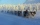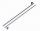At a weather centre, the temperature at midnight was -2 degree Celsius and by noon it had raised 4 degree Celsius. What is the new temperature?

Result

t =  2 °C

Solution:Leave us a comment of this math problem and its solution (i.e. if it is still somewhat unclear...):Be the first to comment!Next similar math problems:

1. Thermometerthermometer showed -12 degrees Celsius at the morning then the temperature rises by 4 degrees and later again increased by 2 degrees at the evening has fallen by 5 degrees and then fall 3 degrees. What end temperature does the thermometer show?
2. This morningThis morning it was 80 degrees F outside the temperature increased 12 degrees F. What is the temperature now?
3. The temperartureThe temperarture is 0 degrees. It drops 5 degrees after 1 hour and then drops 3 degrees more the next hour. What is the temperature after 6 hours?
4. NoontimeIn the morning temperature was 110°F. By noontime it has gone up by 15°F. What was the noon temperature. (Give your answer in °F)
5. Temperature 2Sunday's high temperature was 3 degrees higher than Saturday's. On Monday, the temperature fell 5 degrees, then rose 7 degrees on Tuesday and 4 more on Wednesday. Then it fell 17 degrees to a record low of 31 on Thursday. What was the temperature on Saturd
6. Change in temperatureStarting temperature is 21°C, the highest temperature is 32°C. What is the change in temperature?
7. Roman numerals +Add up the number writtens in Roman numerals. Write the results as a decimal number.
8. Roman numerals 2+Add up the number writtens in Roman numerals. Write the results as a roman numbers.
9. Addition of mixed numeralsAdd two mixed fractions: 2 4/6 + 1 3/6
10. Adding mixed numbersAdd this two mixed numbers: 1 5/6 + 2 2/11=
11. Roses and tulipsAt the florist are 50 tulips and 5 times less roses. How many flowers are in flower shop?
12. Addition of Roman numbersAdded together and write as decimal number: LXVII + MLXIV
13. PlaneOn how many parts divide plane 6 parallels?
14. Foot in busIt was 102 people on the bus. 28 girls had two dogs. A 11 girls had one dog. At the next stop seceded 5 dogs (even with their owners). They got two boys together with three dogs. The bus drove one driver. How many foot were in bus?
15. One frame5 picture frames cost € 12 more than three frames. How much cost one frame?
16. Math classificationIn 3A class are 27 students. One-third got a B in math and the rest got A. How many students received a B in math?
17. CakesOn the bowl were a few cakes. Jane ate one-third of them, Dana ate a quarter of those cakes that remained. a) What part (of the original number of cakes) Dana ate? b) At least how many cakes could be (initially) on thebowl?